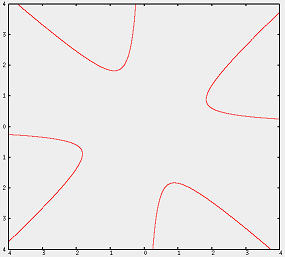#### You may also like### How Many Solutions?

Find all the solutions to the this equation.### Quartics

Investigate the graphs of y = [1 + (x - t)^2][1 + (x + t^)2] as the parameter t varies.### Power Up

Show without recourse to any calculating aid that 7^{1/2} + 7^{1/3} + 7^{1/4} < 7 and 4^{1/2} + 4^{1/3} + 4^{1/4} > 4 . Sketch the graph of f(x) = x^{1/2} + x^{1/3} + x^{1/4} -x

# Maltese Cross

##### Age 16 to 18Challenge Level

Jim sent us his solution:

I made the substitution $xy(x^2 - y^2) = x^2 + y^2$ that was given in the hint. This gave:

$$r^4\cos \theta \sin \theta (\cos^2\theta-\sin^2\theta)= r^2$$

I then subsituted the double angle formulae:

$$\cos2\theta=\cos^2\theta-\sin^2\theta$$ and $$\sin 2\theta=2\sin\theta\cos\theta$$

to get $r^4\sin4\theta=4r^2$

Next, I looked for points distance $2$ from the origin. So $r=2$. This meant that $sin 4\theta=1$, and sosince$\theta$ must be between $0$ and $360$ degrees,it has four solutions: $22.5$ degrees, $112.5$ degrees, $202.5$ degrees and $292.5$ degrees.

I rearranged theformula above to get $\sin4\theta=4/r^2$. Since there are only solutions to $\sin$ that are no more than $1$, $r$ must be at least $2$. This formula also implies that $\sin4\theta$ is never negative, and so $\theta$ can only only take the values in the ranges $0$ to $45$ degrees, $90$ to $135$ degrees, $180$ to $225$ degrees and $270$ to $315$ degrees.

When I made the substitution $y=px$, I got $x^2=\frac{1+p^2}{p(1-p^2)}$. In order for this line to only cut the graph at the origin, this must have no solutions. So we need $x^2< 0$. This meant that $1< p^2$, and so $|p|> 1$ (Not quite right; see amendment below.)

For the next part, I just substituted in the different co-ordinates, and found they satisfy the same equation as $(a,b)$ do, and so must be also on the graph.

Finally, I noticed that from the previous observation, that the graph has rotational symmetry about the origin,with an angle of $90$ degrees. I used this to sketch the graph, which I used a computer to confirm:Deaglan and Ciaran from St Paul's Catholic College agree with most of Jim's reasoning, but have pointed out an error in part C. You can read their amendment here.